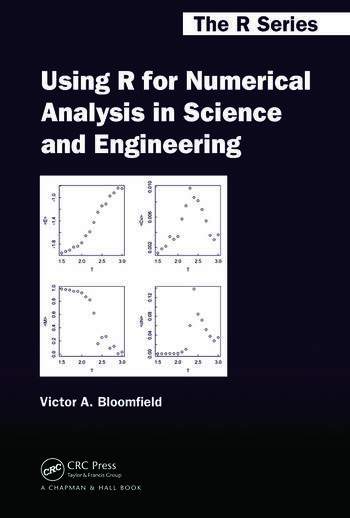# Using R for Numerical Analysis in Science and Engineering

## 1st Edition

Victor A. Bloomfield

Chapman and Hall/CRC
Published April 24, 2014
Reference - 360 Pages - 133 B/W Illustrations
ISBN 9781439884485 - CAT# K13976
Series: Chapman & Hall/CRC The R Series

For Instructors Request Inspection Copy

USD\$110.00

FREE Standard Shipping!

## Preview

### Summary

Instead of presenting the standard theoretical treatments that underlie the various numerical methods used by scientists and engineers, Using R for Numerical Analysis in Science and Engineering shows how to use R and its add-on packages to obtain numerical solutions to the complex mathematical problems commonly faced by scientists and engineers. This practical guide to the capabilities of R demonstrates Monte Carlo, stochastic, deterministic, and other numerical methods through an abundance of worked examples and code, covering the solution of systems of linear algebraic equations and nonlinear equations as well as ordinary differential equations and partial differential equations. It not only shows how to use R’s powerful graphic tools to construct the types of plots most useful in scientific and engineering work, but also:

• Explains how to statistically analyze and fit data to linear and nonlinear models
• Explores numerical differentiation, integration, and optimization
• Describes how to find eigenvalues and eigenfunctions
• Discusses interpolation and curve fitting
• Considers the analysis of time series

Using R for Numerical Analysis in Science and Engineering provides a solid introduction to the most useful numerical methods for scientific and engineering data analysis using R.

#### Instructors

We provide complimentary e-inspection copies of primary textbooks to instructors considering our books for course adoption.